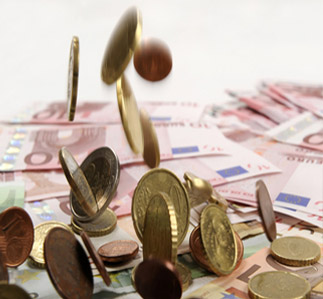# 购汇所持有货币： 人民币(CNY) 美元(USD) —其他货币— 欧元(EUR) 港元(HKD) 日元(JPY) 加元(CAD) 韩元(KRW) 泰铢(THB) 台币(TWD) 英镑(GBP) 荷兰盾(NLG) 澳门元(MOP) 新西兰元(NZD) 新加坡元(SGD) 捷克克朗(CZK) 挪威克郎(NOK) 丹麦克郎(DKK) 德国马克(DEM) 瑞典克朗(SEK) 瑞士法郎(CHF) 印度卢比(INR) 意大利里拉(ITL) 澳大利亚元(AUD) 菲律宾比索(PHP) 比利时法郎(BEF) 俄罗斯卢布(RUB) ——— 乌干达先令(UGX) 罗马尼亚新列伊(RON) 特立尼达多巴哥元(TTD) 圣赫勒拿群岛磅(SHP) 吉尔吉斯斯坦索姆(KGS) 吉布提法郎(DJF) 不丹努扎姆(BTN) 南非兰特(ZAR) 以色列新锡克尔(ILS) 叙利亚磅(SYP) 海地古德(HTG) 也门里亚尔(YER) 乌拉圭比索(UYU) 巴巴多斯元(BBD) 盎司黄金(XAU) 爱沙尼亚克鲁恩(EEK) 芬兰马克(FIM) 马拉维克瓦查(MWK) 印尼盾(IDR) 罗马尼亚列伊(ROL) 巴布亚新几内亚基那(PGK) 斯洛文尼亚托拉尔(SIT) 格林纳达东加勒比元(XCD) 卢旺达法郎(RWF) 尼日利亚奈拉(NGN) 土库曼斯坦马纳特(TMM) 巴哈马元(BSD) 克罗地亚库纳(HRK) 哥伦比亚比索(COP) 乔治亚拉里(GEL) 瓦努阿图瓦图(VUV) 斐济元(FJD) 马尔代夫罗非亚(MVR) 阿塞拜疆曼纳特(AZN) 蒙古图格里克(MNT) 马达加斯加阿里亚里(MGA) 爱尔兰镑(IEP) 苏里南盾(SRG) 科摩罗法郎(KMF) 几内亚法郎(GNF) 所罗门元(SBD) 科威特第纳尔(KWD) 孟加拉塔卡(BDT) 委内瑞拉博利瓦(VEB) 缅元(MMK) 土耳其里拉(TRL) 塔吉克斯坦索莫尼(TJS) 约旦第纳尔(JOD) 巴拿马巴波亚(PAB) 摩尔多瓦列伊(MDL) 佛得角埃斯库多(CVE) 智利比索(CLP) 肯尼亚先令(KES) 苏里南元(SRD) 毛里求斯卢比(MUR) 利比里亚元(LRD) 沙特阿拉伯里亚尔(SAR) 阿根廷比索(ARS) 埃及镑(EGP) 巴拉圭瓜尼(PYG) 土耳其新里拉(TRY) 刚果法郎(CDF) 百慕大元(BMD) 阿曼里亚尔(OMR) 古巴比索(CUP) 尼加拉瓜科多巴(NIO) 冈比亚达拉西(GMD) 斯洛伐克克朗(SKK) 乌兹别克斯坦苏姆(UZS) 赞比亚克瓦查(ZMK) 危地马拉格查尔(GTQ) 尼泊尔卢比(NPR) 纳米比亚元(NAD) 欧元（旧）(XEU) 匈牙利福林(HUF) 老挝基普(LAK) 斯威士兰里兰吉尼(SZL) 沙特阿拉伯里亚尔(UDI) 马耳他里拉(MTL) 文莱币(BND) 坦桑尼亚先令(TZS) 苏丹镑(SDG) 莱索托洛蒂(LSL) 开曼群岛币(KYD) 斯里兰卡卢比(LKR) 马其顿第纳尔(MKD) 墨西哥比索(MXN) 加纳塞第(GHC) 冰岛克郎(ISK) 利比亚第纳尔(LYD) 塞拉里昂利昂(SLL) 巴基斯坦卢比(PKR) 安第列斯群岛盾(ANG) 塞舌尔卢比(SCR) 奥地利先令(ATS) 黎巴嫩镑(LBP) 阿联酋迪拉姆(AED) 新加纳塞第(GHS) 玻利维亚币(BOB) 厄立特里亚纳克法(ERN) 直布罗陀镑(GIP) 卡塔尔里亚尔(QAR) 巴林第纳尔(BHD) 伊朗里亚尔(IRR) 博茨瓦纳普拉(BWP) 洪都拉斯伦皮拉(HNL) 阿尔巴尼亚列克(ALL) 赛尔维亚第纳尔(RSD) 马来西亚林吉特(MYR) 埃塞俄比亚比尔(ETB) 圣多美和普林西比多布拉(STD) 安道尔西班牙银币(ADP) 保加利亚列弗(BGN) 多米尼加比索(DOP) 亚美尼亚打兰(AMD) 法国太平洋法郎(XPF) 特别提款权(SDR) 牙买加元(JMD) 毛里塔尼亚乌吉亚(MRO) 加那利群岛比塞塔(ESP) 津巴布韦元(ZWD) 拉脱维亚拉茨(LVL) 布隆迪法郎(BIF) 马提尼克法郎(FRF) 突尼斯第纳尔(TND) 厄瓜多尔苏克雷(ECS) 越南盾(VND) 希腊德拉克马(GRD) 秘鲁新索尔(PEN) 阿尔及利亚第纳尔(DZD) 莫桑比克梅蒂卡尔(MZN) 阿鲁巴弗罗林(AWG) 莫桑比克美提卡(MZM) 多哥非共体法郎(XOF) 葡萄牙埃斯库多(PTE) 哈萨克斯坦坚戈(KZT) 乌克兰赫夫米(UAH) 伯利兹元(BZD) 波斯尼亚(BAM) 摩洛哥迪拉姆(MAD) 白俄罗斯卢布(BYR) 立陶宛立特(LTL) 柬埔寨瑞尔(KHR) 西非法郎(XAF) 塞浦路斯镑(CYP) 圭亚那元(GYD) 巴西雷亚尔(BRL) 阿富汗尼(AFN) 哥斯达黎加科朗(CRC) 萨尔瓦多科朗(SVC) 伊拉克第纳尔(IQD) 波兰兹罗提(PLN) 索马里先令(SOS) 汤加潘加(TOP) 安哥拉宽扎(AOA) 卢森堡法郎(LUF) 朝鲜元(KPW) 兑换的货币： 美元(USD) 人民币(CNY) —其他货币— 欧元(EUR) 港元(HKD) 日元(JPY) 加元(CAD) 韩元(KRW) 泰铢(THB) 台币(TWD) 英镑(GBP) 荷兰盾(NLG) 澳门元(MOP) 新西兰元(NZD) 新加坡元(SGD) 捷克克朗(CZK) 挪威克郎(NOK) 丹麦克郎(DKK) 德国马克(DEM) 瑞典克朗(SEK) 瑞士法郎(CHF) 印度卢比(INR) 意大利里拉(ITL) 澳大利亚元(AUD) 菲律宾比索(PHP) 比利时法郎(BEF) 俄罗斯卢布(RUB) ——— 乌干达先令(UGX) 罗马尼亚新列伊(RON) 特立尼达多巴哥元(TTD) 圣赫勒拿群岛磅(SHP) 吉尔吉斯斯坦索姆(KGS) 吉布提法郎(DJF) 不丹努扎姆(BTN) 南非兰特(ZAR) 以色列新锡克尔(ILS) 叙利亚磅(SYP) 海地古德(HTG) 也门里亚尔(YER) 乌拉圭比索(UYU) 巴巴多斯元(BBD) 盎司黄金(XAU) 爱沙尼亚克鲁恩(EEK) 芬兰马克(FIM) 马拉维克瓦查(MWK) 印尼盾(IDR) 罗马尼亚列伊(ROL) 巴布亚新几内亚基那(PGK) 斯洛文尼亚托拉尔(SIT) 格林纳达东加勒比元(XCD) 卢旺达法郎(RWF) 尼日利亚奈拉(NGN) 土库曼斯坦马纳特(TMM) 巴哈马元(BSD) 克罗地亚库纳(HRK) 哥伦比亚比索(COP) 乔治亚拉里(GEL) 瓦努阿图瓦图(VUV) 斐济元(FJD) 马尔代夫罗非亚(MVR) 阿塞拜疆曼纳特(AZN) 蒙古图格里克(MNT) 马达加斯加阿里亚里(MGA) 爱尔兰镑(IEP) 苏里南盾(SRG) 科摩罗法郎(KMF) 几内亚法郎(GNF) 所罗门元(SBD) 科威特第纳尔(KWD) 孟加拉塔卡(BDT) 委内瑞拉博利瓦(VEB) 缅元(MMK) 土耳其里拉(TRL) 塔吉克斯坦索莫尼(TJS) 约旦第纳尔(JOD) 巴拿马巴波亚(PAB) 摩尔多瓦列伊(MDL) 佛得角埃斯库多(CVE) 智利比索(CLP) 肯尼亚先令(KES) 苏里南元(SRD) 毛里求斯卢比(MUR) 利比里亚元(LRD) 沙特阿拉伯里亚尔(SAR) 阿根廷比索(ARS) 埃及镑(EGP) 巴拉圭瓜尼(PYG) 土耳其新里拉(TRY) 刚果法郎(CDF) 百慕大元(BMD) 阿曼里亚尔(OMR) 古巴比索(CUP) 尼加拉瓜科多巴(NIO) 冈比亚达拉西(GMD) 斯洛伐克克朗(SKK) 乌兹别克斯坦苏姆(UZS) 赞比亚克瓦查(ZMK) 危地马拉格查尔(GTQ) 尼泊尔卢比(NPR) 纳米比亚元(NAD) 欧元（旧）(XEU) 匈牙利福林(HUF) 老挝基普(LAK) 斯威士兰里兰吉尼(SZL) 沙特阿拉伯里亚尔(UDI) 马耳他里拉(MTL) 文莱币(BND) 坦桑尼亚先令(TZS) 苏丹镑(SDG) 莱索托洛蒂(LSL) 开曼群岛币(KYD) 斯里兰卡卢比(LKR) 马其顿第纳尔(MKD) 墨西哥比索(MXN) 加纳塞第(GHC) 冰岛克郎(ISK) 利比亚第纳尔(LYD) 塞拉里昂利昂(SLL) 巴基斯坦卢比(PKR) 安第列斯群岛盾(ANG) 塞舌尔卢比(SCR) 奥地利先令(ATS) 黎巴嫩镑(LBP) 阿联酋迪拉姆(AED) 新加纳塞第(GHS) 玻利维亚币(BOB) 厄立特里亚纳克法(ERN) 直布罗陀镑(GIP) 卡塔尔里亚尔(QAR) 巴林第纳尔(BHD) 伊朗里亚尔(IRR) 博茨瓦纳普拉(BWP) 洪都拉斯伦皮拉(HNL) 阿尔巴尼亚列克(ALL) 赛尔维亚第纳尔(RSD) 马来西亚林吉特(MYR) 埃塞俄比亚比尔(ETB) 圣多美和普林西比多布拉(STD) 安道尔西班牙银币(ADP) 保加利亚列弗(BGN) 多米尼加比索(DOP) 亚美尼亚打兰(AMD) 法国太平洋法郎(XPF) 特别提款权(SDR) 牙买加元(JMD) 毛里塔尼亚乌吉亚(MRO) 加那利群岛比塞塔(ESP) 津巴布韦元(ZWD) 拉脱维亚拉茨(LVL) 布隆迪法郎(BIF) 马提尼克法郎(FRF) 突尼斯第纳尔(TND) 厄瓜多尔苏克雷(ECS) 越南盾(VND) 希腊德拉克马(GRD) 秘鲁新索尔(PEN) 阿尔及利亚第纳尔(DZD) 莫桑比克梅蒂卡尔(MZN) 阿鲁巴弗罗林(AWG) 莫桑比克美提卡(MZM) 多哥非共体法郎(XOF) 葡萄牙埃斯库多(PTE) 哈萨克斯坦坚戈(KZT) 乌克兰赫夫米(UAH) 伯利兹元(BZD) 波斯尼亚(BAM) 摩洛哥迪拉姆(MAD) 白俄罗斯卢布(BYR) 立陶宛立特(LTL) 柬埔寨瑞尔(KHR) 西非法郎(XAF) 塞浦路斯镑(CYP) 圭亚那元(GYD) 巴西雷亚尔(BRL) 阿富汗尼(AFN) 哥斯达黎加科朗(CRC) 萨尔瓦多科朗(SVC) 伊拉克第纳尔(IQD) 波兰兹罗提(PLN) 索马里先令(SOS) 汤加潘加(TOP) 安哥拉宽扎(AOA) 卢森堡法郎(LUF) 朝鲜元(KPW) 需兑换数量：